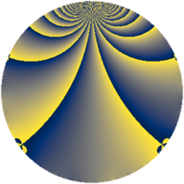# Properties

 Label 966.2.jLevel $966$ Weight $2$ Character orbit 966.j Rep. character $\chi_{966}(137,\cdot)$ Character field $\Q(\zeta_{6})$ Dimension $128$ Newform subspaces $1$ Sturm bound $384$ Trace bound $0$

# Related objects

## Defining parameters

 Level: $$N$$ $$=$$ $$966 = 2 \cdot 3 \cdot 7 \cdot 23$$ Weight: $$k$$ $$=$$ $$2$$ Character orbit: $$[\chi]$$ $$=$$ 966.j (of order $$6$$ and degree $$2$$) Character conductor: $$\operatorname{cond}(\chi)$$ $$=$$ $$483$$ Character field: $$\Q(\zeta_{6})$$ Newform subspaces: $$1$$ Sturm bound: $$384$$ Trace bound: $$0$$

## Dimensions

The following table gives the dimensions of various subspaces of $$M_{2}(966, [\chi])$$.

Total New Old
Modular forms 400 128 272
Cusp forms 368 128 240
Eisenstein series 32 0 32

## Trace form

 $$128q + 64q^{4} + 8q^{6} - 4q^{9} + O(q^{10})$$ $$128q + 64q^{4} + 8q^{6} - 4q^{9} - 64q^{16} + 4q^{24} - 72q^{25} + 24q^{27} - 8q^{36} + 4q^{39} + 12q^{46} + 72q^{49} + 4q^{54} - 96q^{55} - 128q^{64} - 56q^{69} + 32q^{70} - 40q^{73} - 40q^{75} - 16q^{78} + 36q^{81} - 32q^{82} - 64q^{85} - 24q^{87} + 32q^{93} - 8q^{94} - 4q^{96} + O(q^{100})$$

## Decomposition of $$S_{2}^{\mathrm{new}}(966, [\chi])$$ into newform subspaces

Label Dim. $$A$$ Field CM Traces $q$-expansion
$$a_2$$ $$a_3$$ $$a_5$$ $$a_7$$
966.2.j.a $$128$$ $$7.714$$ None $$0$$ $$0$$ $$0$$ $$0$$

## Decomposition of $$S_{2}^{\mathrm{old}}(966, [\chi])$$ into lower level spaces

$$S_{2}^{\mathrm{old}}(966, [\chi]) \cong$$ $$S_{2}^{\mathrm{new}}(483, [\chi])$$$$^{\oplus 2}$$